### 资讯专栏INFORMATION COLUMNalogy / 1690人阅读

1.集合里的元素类型不一定相同。
2.集合里的元素没有顺序。

# 数组

1.数组会用一些名为索引的数字来标识每项数据在数组中的位置，且在大多数编程语言中，索引是从 0 算起的。我们可以根据数组中的索引，快速访问数组中的元素。列表中没有索引，这是数组与列表最大的不同点。
2.数组中的元素在内存中是连续存储的，且每个元素占用相同大小的内存。列表中的元素在内存中可能彼此相邻，也可能不相邻。比如列表的另一种实现方式——链表，它的元素在内存中则不一定是连续的

# 1.Leetcode724.寻找数组的中心下标

``class Solution {public:    int pivotIndex(vector<int>& nums) {        int a;        memset(a,0,sizeof a);        int len=nums.size();        for(int i=1;i<=len;i++){                     a[i]=a[i-1]+nums[i-1];        }                for(int i=1;i<=len;i++){            if(a[i-1]==a[len]-a[i]){                return i-1;            }        }        return -1;    }};``

# 2.Leetcode35.搜索插入位置

``class Solution {public:    int searchInsert(vector<int>& nums, int target) {        int len=nums.size();        int l=0,r=len-1;        while(l<r){            int mid=(l+r)/2;            if(nums[mid]>=target){                r=mid;            }else{                l=mid+1;            }        }        if(l==len-1&&target>nums[l])return l+1;        return l;    }};``

# 3.Leetcode56.合并区间

``const int INF=-0x3f3f3f3f;class Solution {public:    vector<vector<int>> merge(vector<vector<int>>& intervals) {        vector<vector<int>>ans;        sort(intervals.begin(),intervals.end());        int len=intervals.size();        int begin,end;         for(int i=0;i<len;i++){             vector<int>line;             int a=intervals[i],b=intervals[i];             if(!i){                 begin=a;                 end=b;             }             if(i&&a>end){                line.push_back(begin);                line.push_back(end);                ans.push_back(line);                begin=a;                end=b;                }else if(i&&a<=end){                 end=max(end,b);             }             if(i==len-1){                 vector<int>tail;                 tail.push_back(begin);                 tail.push_back(end);                  ans.push_back(tail);             }                                   }         return ans;    }};``

# 4.面试题 01.07. 旋转矩阵

``class Solution {public:    void rotate(vector<vector<int>>& matrix) {        int len=matrix.size();               for(int i=0;i<len;i++){            for(int j=0;j<i;j++){                swap(matrix[i][j],matrix[j][i]);            }        }                for(int i=0;i<len;i++){            for(int j=0;j<len/2;j++){                swap(matrix[i][j],matrix[i][len-j-1]);            }        }                 }};``

# 5.面试题 01.08. 零矩阵

``class Solution {public:    void setZeroes(vector<vector<int>>& matrix) {        int n=matrix.size();        int m=matrix.size();        bool row=false,col=false;        for(int i=0;i<m;i++){            if(!matrix[i]){                row=true;                break;            }        }        for(int i=0;i<n;i++){            if(!matrix[i]){                col=true;                break;            }        }        for(int i=1;i<n;i++){            for(int j=1;j<m;j++){                if(!matrix[i][j])matrix[j]=matrix[i]=0;            }        }        for(int i=1;i<n;i++){            for(int j=1;j<m;j++){                if(!matrix[j]||!matrix[i])matrix[i][j]=0;            }        }        if(row){            for(int i=0;i<m;i++){                matrix[i]=0;            }        }         if(col){            for(int i=0;i<n;i++){                matrix[i]=0;            }        }    }};``

``class Solution {public:    void setZeroes(vector<vector<int>>& matrix) {        int n=matrix.size();        int m=matrix.size();        bool col=false;        //注意先列再行不然行matrix的改变会影响到列        for(int i=0;i<n;i++){            if(!matrix[i]){                col=true;                break;            }        }        for(int i=0;i<m;i++){            if(!matrix[i]){                matrix=0;                break;            }        }                for(int i=1;i<n;i++){            for(int j=1;j<m;j++){                if(!matrix[i][j])matrix[j]=matrix[i]=0;            }        }        for(int i=1;i<n;i++){            for(int j=1;j<m;j++){                if(!matrix[j]||!matrix[i])matrix[i][j]=0;            }        }        if(matrix==0){            for(int i=0;i<m;i++){                matrix[i]=0;            }        }         if(col){            for(int i=0;i<n;i++){                matrix[i]=0;            }        }    }};``

# 6.Leetcode498. 对角线遍历

``class Solution {public:    vector<int> findDiagonalOrder(vector<vector<int>>& mat) {          vector<int>ans;          int n=mat.size(),m=mat.size();               bool flag=true;          int pre;          pre=0;          int len=m+n;          for(int i=0;i<len*2-1;i++){             if(flag){                 int row=pre;                 int col=i-pre;                  int b=2*len-i;                     while(!(row>=0&&row<n&&col>=0&&col<m)&&b--){                   row--;                   col++;                              }                     while(row>=0&&row<n&&col>=0&&col<m){                     ans.push_back(mat[row--][col++]);                 }                               pre=col;             }else{                 int row=i-pre;                 int col=pre;                 int b=2*len-1-i;                 while(!(row>=0&&row<n&&col>=0&&col<m)&&b--){                    row++;                    col--;                 }                 while(row>=0&&row<n&&col>=0&&col<m){                     ans.push_back(mat[row++][col--]);                 }                 pre=row;             }             flag=!flag;          }                   return ans;    }};``

# 7.Leetcode14. 最长公共前缀

``class Solution {public:    string longestCommonPrefix(vector<string>& strs) {         string ans;         int len=strs.size();         int n=strs.size();         for(int i=0;i<n;i++){            auto c=strs[i];            int j;           for(j=1;j<len;j++){              if(c!=strs[j][i])break;           }           if(j==len){               ans+=c;           }else{               return ans;           }         }         return ans;    }};``

``class Solution {public:    string longestCommonPrefix(vector<string>& strs) {         string ans;         sort(strs.begin(),strs.end());         string st=strs,end=strs[strs.size()-1];         int len1=st.size(),len2=end.size();         int t=0;         while(t<len1&&t<len2&&st[t]==end[t]){             ans+=st[t];             t++;         }         return ans;    }};``

# 8.Leetcode5. 最长回文子串

``````class Solution {public:    string longestPalindrome(string s) {       int len=s.size();       if(len<2

私有云

云服务器

数组和字符串
php数组和字符串
js中字符和数组
字符串算法题

文章版权归作者所有，未经允许请勿转载,若此文章存在违规行为，您可以联系管理员删除。

转载请注明本文地址：https://www.ucloud.cn/yun/123292.html

上一篇：stm32mini开发板lora模块例程中中文字库更新失败的解决方案

下一篇：【解题报告】《算法零基础100讲》(第25讲) 字符串算法(五) - 字符串反转

``````
``` 相关文章 Leetcode打卡——二叉搜索树（共8题） ...值必须小于（或等于）存储在其右子树中的任何值。 1.Leetcode98. 验证二叉搜索树 法一：利用二叉树的性质，根结点的值大于左子树上的点，小于右子树上的点 const long long MAX=2e31;const long long MIN=-MAX;class Solution {public: bool isValid...Olivia 2021-11-15 11:38 评论0 收藏0 【万人千题】结对编程排位赛(第一期) 第二周 排名公布，冠军成功卫冕，啊这…… ...」、「 白银 」、「 青铜 」。记录下每个人的 CSDN博客、LeetCode 主页，排名靠前的人可以得到更多的曝光。 ?【万人千题】结对编程排位赛?   如果想参加的「 第二期 」的同学，可以先联系作者加群，看看第一期的同袍...PumpkinDylan 2021-11-22 09:34 评论0 收藏0 【万人千题】大学生算法社区火爆开启，每日打卡学习，诚邀妳的加入 ...入门100例》(第10例) 平均数 《算法零基础100讲》(第22讲) 字符串 前言   给自己树立一个「 目标 」是非常重要的，有「 目标 」才会有「 方向 」，有「 目标 」才会有「 动力 」，有「 目标 」才会有「 人生的意义 」。有了...morgan 2021-11-12 10:36 评论0 收藏0 LeetCode 之 JavaScript 解答第150题 —— 逆波兰表达式求值 ...法写的全面还是需要考虑到很多方面的。1）数组中的是字符串类型，要进行数据类型转换 parseInt() 。 2）两个操作数进行运算时，第二个出栈的操作数在前，第一个出栈的操作数在后（注意除法）。 3）对于浮点型数据，只取小...104828720 2019-08-23 16:56 评论0 收藏0 算法刷题打卡（十） ... 56 合并区间 56. 合并区间 - 力扣（LeetCode） (leetcode-cn.com) 以数组 intervals 表示若干个区间的集合，其中单个区间为 intervals[i] = [starti, endi] 。请你合并所有重叠的区间，并返回一个不重叠的区间数组，该数组...BigTomato 2021-11-23 09:51 评论0 收藏0 发表评论 登陆后可评论 0条评论 ```
```alogy 男|高级讲师 我要关注 我要私信 TA的文章 阅读更多 磁盘管理与文件系统 阅读 2266·2021-11-25 09:43 pacificrack跑路了!如有站长在使用请转移备份数据! 阅读 1539·2021-11-18 13:25 Leetcode打卡——数组和字符串（共22题） 阅读 1691·2021-11-15 11:38 python之pil的使用 阅读 3039·2021-10-14 09:43 获取域管理员权限的几种方式 阅读 1490·2021-10-14 09:42 无主机号码怎么回事-手机发信息显示无号码怎么回事？ 阅读 2334·2021-09-22 15:52 怎么计算网络号哥和主机号-如何计算IP地址的网络号和主机号？ 阅读 1108·2021-09-22 15:49 开发一个自己的 CSS 框架（四） 阅读 1843·2019-08-30 15:54 云社区相关服务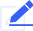提问学习认证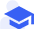产品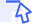技术服务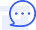售前咨询 最新活动\$(function(){ \$.ajax({ type: "GET", url:"https://www.ucloud.cn/yun/ad/getad/1.html", cache: false, success: function(text){ \$(".adhtml").html(text); } }); }) ```
``` ```
``` ```
``` 阅读需要支付1元查看 var prei=0; \$(".site-seo-depict pre").each(function(){ var html=\$(this).html().replace("<code>","").replace("</code>","").replace('<code class="javascript hljs" codemark="1">',''); \$(this).attr('data-clipboard-text',html).attr("id","pre"+prei); \$(this).html("").append("<code>"+html+"</code>"); prei++; }) \$(".site-seo-depict img").each(function(){ if(\$(this).attr("src").indexOf('data:image/svg+xml')!= -1){ \$(this).remove(); } }) \$("LINK[href*='style-49037e4d27.css']").remove(); \$("LINK[href*='markdown_views-d7a94ec6ab.css']").remove(); layui.use(['jquery', 'layer','code'], function(){ \$("pre").attr("class","layui-code"); \$("pre").attr("lay-title",""); \$("pre").attr("lay-skin",""); layui.code(); \$(".layui-code-h3 a").attr("class","copycode").html("复制代码 ").attr("onclick","copycode(this)"); }); function copycode(target){ var id=\$(target).parent().parent().attr("id"); var clipboard = new ClipboardJS("#"+id); clipboard.on('success', function(e) { e.clearSelection(); alert("复制成功") }); clipboard.on('error', function(e) { alert("复制失败") }); } //\$(".site-seo-depict").html(\$(".site-seo-depict").html().slice(0, -5)); hljs.initHighlightingOnLoad(); function setcode(){ var _html=''; document.querySelectorAll('pre code').forEach((block) => { var _tmptext=\$.trim(\$(block).text()); if(_tmptext!=''){ _html=_html+_tmptext; console.log(_html); } }); } function payread(){ layer.open({ type: 1, title:"付费阅读", shadeClose: true, content: \$('#payread') }); } // 举报 function jupao_tip(){ layer.open({ type: 1, title:false, shadeClose: true, content: \$('#jubao') }); } \$(".getcommentlist").click(function(){ var _id=\$(this).attr("dataid"); var _tid=\$(this).attr("datatid"); \$("#articlecommentlist"+_id).toggleClass("hide"); var flag=\$("#articlecommentlist"+_id).attr("dataflag"); if(flag==1){ flag=0; }else{ flag=1; //加载评论 loadarticlecommentlist(_id,_tid); } \$("#articlecommentlist"+_id).attr("dataflag",flag); }) \$(".add-comment-btn").click(function(){ var _id=\$(this).attr("dataid"); \$(".formcomment"+_id).toggleClass("hide"); }) \$(".btn-sendartcomment").click(function(){ var _aid=\$(this).attr("dataid"); var _tid=\$(this).attr("datatid"); var _content=\$.trim(\$(".commenttext"+_aid).val()); if(_content==''){ alert("评论内容不能为空"); return false; } var touid=\$("#btnsendcomment"+_aid).attr("touid"); if(touid==null){ touid=0; } addarticlecomment(_tid,_aid,_content,touid); }) \$(".button_agree").click(function(){ var supportobj = \$(this); var tid = \$(this).attr("id"); \$.ajax({ type: "GET", url:"https://www.ucloud.cn/yun/index.php?topic/ajaxhassupport/" + tid, cache: false, success: function(hassupport){ if (hassupport != '1'){ \$.ajax({ type: "GET", cache:false, url: "https://www.ucloud.cn/yun/index.php?topic/ajaxaddsupport/" + tid, success: function(comments) { supportobj.find("span").html(comments+"人赞"); } }); }else{ alert("您已经赞过"); } } }); }); function attenquestion(_tid,_rs){ \$.ajax({ //提交数据的类型 POST GET type:"POST", //提交的网址 url:"https://www.ucloud.cn/yun/favorite/topicadd.html", //提交的数据 data:{tid:_tid,rs:_rs}, //返回数据的格式 datatype: "json",//"xml", "html", "script", "json", "jsonp", "text". //在请求之前调用的函数 beforeSend:function(){}, //成功返回之后调用的函数 success:function(data){ var data=eval("("+data+")"); console.log(data) if(data.code==2000){ layer.msg(data.msg,function(){ if(data.rs==1){ //取消收藏 \$(".layui-layer-tips").attr("data-tips","收藏文章"); \$(".layui-layer-tips").html('<i class="fa fa-heart-o"></i>'); } if(data.rs==0){ //收藏成功 \$(".layui-layer-tips").attr("data-tips","已收藏文章"); \$(".layui-layer-tips").html('<i class="fa fa-heart"></i>') } }) }else{ layer.msg(data.msg) } } , //调用执行后调用的函数 complete: function(XMLHttpRequest, textStatus){ postadopt=true; }, //调用出错执行的函数 error: function(){ //请求出错处理 postadopt=false; } }); }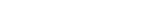UCloud （优刻得科技股份有限公司）是中立、安全的云计算服务平台，坚持中立，不涉足客户业务领域。公司自主研发IaaS、PaaS、大数据流通平台、AI服务平台等一系列云计算产品，并深入了解互联网、传统企业在不同场景下的业务需求，提供公有云、混合云、私有云、专有云在内的综合性行业解决方案。 UCloud与云服务 公司介绍 加入我们 UCan线上公开课 行业解决方案 产品动态 热门产品 CDN 数字孪生 私有云 云手机 GPU服务器 物理机租用 服务器托管 高防服务器实时音视频 社区栏目 专栏文章 专业问答 云学院 专题地图 常见问题 安全中心 新闻动态 媒体动态 客户案例 公告扫扫了解更多 China CNC Milling vps linux cheap Copyright © 2012-2023 UCloud 优刻得科技股份有限公司｜沪公网安备 31011002000058号｜ 沪ICP备12020087号-3｜ var _hmt = _hmt || []; (function() { var hm = document.createElement("script"); hm.src = "https://hm.baidu.com/hm.js?290c2650b305fc9fff0dbdcafe48b59d"; var s = document.getElementsByTagName("script"); s.parentNode.insertBefore(hm, s); })(); window.dataLayer = window.dataLayer || []; function gtag(){dataLayer.push(arguments);} gtag('js', new Date()); gtag('config', 'G-DZSMXQ3P9N'); (function(){ var el = document.createElement("script"); el.src = "https://lf1-cdn-tos.bytegoofy.com/goofy/ttzz/push.js?99f50ea166557aed914eb4a66a7a70a4709cbb98a54ecb576877d99556fb4bfc3d72cd14f8a76432df3935ab77ec54f830517b3cb210f7fd334f50ccb772134a"; el.id = "ttzz"; var s = document.getElementsByTagName("script"); s.parentNode.insertBefore(el, s); })(window) < \$(".site-seo-depict *,.site-content-answer-body *,.site-body-depict *").css("max-width","100%"); ```